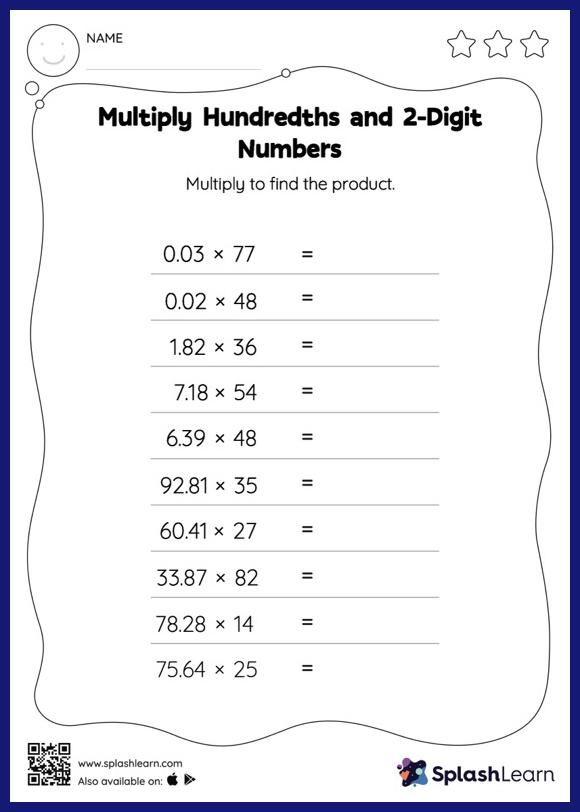# Multiply Hundredths and 2-Digit Numbers: Horizontal Multiplication Worksheet

Home > Multiply Hundredths and 2-Digit Numbers: Horizontal MultiplicationThis multiply hundredths and 2-digit numbers worksheet consists of tasks to help your young mathematician develop fluency with multiplication. When multiplying a decimal by a two-digit number, students may ignore the decimal point during calculations and bring it back when writing the result. This multiply hundredths and 2-digit numbers worksheet provides plenty of practice with this concept. In this worksheet, students practice solving problems written in the horizontal format. How numbers are laid out in a problem affects the method a student employs to solve it. Therefore to develop actual fluency and mastery of multiple strategies, students must practice different formats.